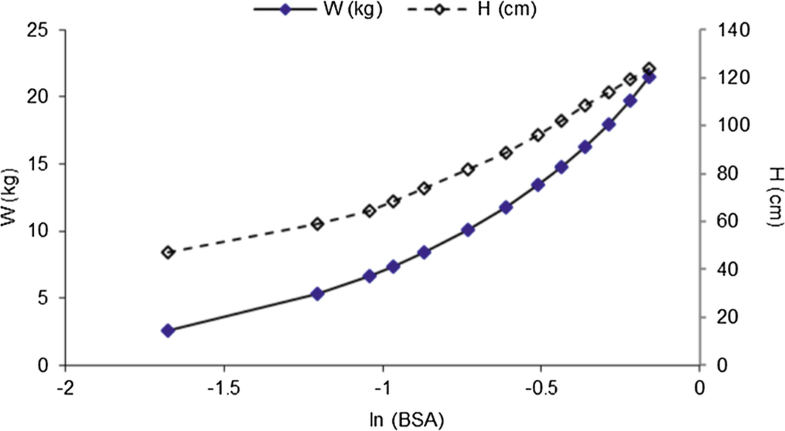# Dosage Calculations Based on Body Surface Area (BSA)

• Michalakis Savva
Chapter

## Abstract

In the previous chapter, drug concentration in the blood was correlated to the clearance and drug distribution in the body. In this chapter, I discuss the relation of the body surface area (BSA) to the body weight. Subsequently, a number of complicated BSA equations are derived by applying simple concepts of geometry. Through these derivations you have the opportunity to comprehend and further apply the equations to calculate drug doses. The chapter is focused on the rational use of BSA equations for dosage calculations. Close to the end of the chapter, higher exponential equations are solved, either as they are or after conversion to the more convenient linear form to calculate doses for pediatric populations. In general, not all the drugs can be dosed using the concept of body surface area. Clinicians have to be able to see the connection between drug disposition, organ size, and body size in order to confidently use these equations to calculate doses. This chapter offers a conceptual approach to the use of BSA equations for dosage calculations. It also discusses the advantages and disadvantages of the method.

## Keywords

Body surface area Dubois and Dubois’s formula Boyd’s formula Fujimoto’s formula Haycock’s formula Mosteller’s formula

## Supplementary material

Open image in new windowLesson 14.1

Dosage calculations based on BSA. Description: (a) Body weight-based dosing versus BSA-based dosing strategies. (b) BSA calculations from height and weight using equations and nomograms. (c) Dosage regimens using BSA. (d) When to apply BSA-based dosage regimens (MP4 1086931 kb)

## References

1. T. Kouno, N. Katsumata, H. Mukai, M. Ando, T. Watanabe, Standardization of the Body Surface Area (BSA) formula to calculate the dose of anticancer agents in Japan. Jpn. J. Clin. Oncol. 33, 309–313 (2006)
2. Y.-X. Yang, C.-Y. Yu, W.-K. Chiou, The 3D scanner for measuring body surface area: a simplified calculation in the Chinese adult. Appl. Ergon. 34, 273–278 (2003)